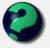Discussion about math, puzzles, games and fun.   Useful symbols: ÷ × ½ √ ∞ ≠ ≤ ≥ ≈ ⇒ ± ∈ Δ θ ∴ ∑ ∫  π  -¹ ² ³ °

You are not logged in.

## #1 2010-08-15 12:58:53

boy15
Member
Registered: 2010-03-29
Posts: 16

### Discrete math help

Hi, I need help on a problem from my discrete math textbook.

If

is a polynomial with integer coefficients, and that this polynomial takes the value zero at four distinct integer values a, b, c and d.

Show that there exists no integer value n for which

.

I don't know how to do it and my book doesn't go through it well enough. Can anyone help me?

Thanks

Offline

## #2 2010-08-16 00:25:01

mathsyperson
ModeratorRegistered: 2005-06-22
Posts: 4,900

### Re: Discrete math help

I think the key to this is that the polynomial has factors of (x-a), (x-b), (x-c), (x-d).

13 only has two factors - 1 and 13 - but here we've shown that any number produced using this polynomial will have at least 4.
(The exception being when you use a value of n that causes P(n) = 0, but P(n) isn't 13 in that case either)

Why did the vector cross the road?
It wanted to be normal.

Offline

## #3 2010-08-16 09:33:26

bob bundyRegistered: 2010-06-20
Posts: 8,462

### Re: Discrete math help

You have been given that

P(x) = (x-a)(x-b)(x-c)(x-d)Q(x) for a, b, c, d all integers and Q is some polynomial of lower power than P.

Now assume 'to the contrary' that n exists,

ie  P(x) = (x-n)R(x) + 13   where R is also a polynomial of lower order than P

Put x = n in both expressions

(n-a)(n-b)(n-c)(n-d)Q(n) = 0 + 13

This is a contradiction as 13 cannot have these many (distinct) integer factors, so the assumption was false.

Hope this helps,

Bob

Last edited by bob bundy (2010-08-16 09:35:24)

Children are not defined by school ...........The Fonz
You cannot teach a man anything;  you can only help him find it within himself..........Galileo Galilei
Sometimes I deliberately make mistakes, just to test you!  …………….Bob BundyOffline

## #4 2010-08-17 01:23:33

bob bundyRegistered: 2010-06-20
Posts: 8,462

### Re: Discrete math help

Hi again,

In the middle of the night I had a further thought and I now think the last lines above need this addition.

(n-a)(n-b)(n-c)(n-d)Q(n) = 0 + 13

It is possible to choose 'n' and 'a' so that (n-a) = 13 (say) and 'b' so that (n-b) = 1 and 'c' so that (n-c) = -1 and Q so that Q(n) = -1, but, as a, b, c, d are said to be distinct, there is no other factor of 13 in the set of integers for (n-d) and therefore ....

This is a contradiction as 13 cannot have these many (distinct) integer factors, so the assumption was false.

Children are not defined by school ...........The Fonz
You cannot teach a man anything;  you can only help him find it within himself..........Galileo Galilei
Sometimes I deliberately make mistakes, just to test you!  …………….Bob BundyOffline

## #5 2010-08-22 11:46:37

boy15
Member
Registered: 2010-03-29
Posts: 16

### Re: Discrete math help

Thanks all, I understand now.

Offline

## #6 2010-08-22 23:14:42

bob bundyRegistered: 2010-06-20
Posts: 8,462

### Re: Discrete math help

You're welcome

Bob

Children are not defined by school ...........The Fonz
You cannot teach a man anything;  you can only help him find it within himself..........Galileo Galilei
Sometimes I deliberately make mistakes, just to test you!  …………….Bob BundyOffline